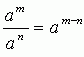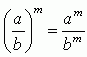Name: ___________________Date:___________________

 Email us to get an instant 20% discount on highly effective K-12 Math & English kwizNET Programs!

### High School Mathematics - 21.16 Properties of Exponents

 Laws of exponents: am x an = am+n (am)n = amn (ab)m = am x bma0 = 1 a1 = a a-n = 1/an Direction: Solve the following problems. Also write the laws of exponents on a piece of paper.Name: ___________________Date:___________________

### High School Mathematics - 21.16 Properties of Exponents

 Q 1: Simplify, x2.9x3 = _____?(9x)59x5 Q 2: Simplify, 4x2.2x3 = _____?8x5(8x)5 Q 3: Find the value of 3(2x-1), when x=9?5145 Q 4: Simplify, [(2x)2]2 = _____?16x216x4 Q 5: Write 4.x.x.x.y.y in exponent form?4(xy)34x3y2 Q 6: Write a.a.a in exponent form?a33a Q 7: Find the value of a7 when a=1?71 Q 8: Simplify, [(x)7]2 = _____?x7x14 Question 9: This question is available to subscribers only! Question 10: This question is available to subscribers only!